Lecture 25: Consensus & Beyond

Reminders

Final Project:

• Short video due today
• Final submission next Friday, May 28, 5pm Eastern

All submissions to Google drive folder

Last Time

Consensus

• $n$ processes, each with private input
• some processes may crash
• must produce output satisfying following properties
• Agreement: all processes output the same value
• Validity: if all systems have the same input, they all output that value
• Termination: all (non-faulty) processes decide on an output and terminate after a finite number of steps

Our Goal

Theorem (FLP, 1985). There is no algorithm that achieves consensus in the presence of even a single faulty process.

• Assumes atomic read/write shared memory
• Special case: there is no wait-free protocol for consensus for any $n > 1$
• wait-free is stronger assumption than termination
• Consider binary consensus all inputs 0/1

Also Last Time

Lemma 2. Suppose $A$ solves consensus. Then there is a bivalent initial state.

• Recall a bivalent execution (or initial state) is an execution from which the output could be 0 or 1 (depending or scheduler)

Lemma 3. Every consensus protocol has a critical execution.

• $E$ is a critical execution if it is bivalent, but every extension of $E$ is univalent
• any process taking a single step from $E$ determines the output

These properties hold for all consensus protocols

Today

Finish the proof of FLP

Outline of Proof of FLP

Assume only 2 processes, $P_0$ and $P_1$

1. Start from a critical execution $E$
• $E$ is bivalent, but any extension is univalent
2. Consider all possibilities for next step:
3. Show that in any case, we contradict either criticality of E or correctness of protocol

Assumptions

Without loss of generality:

1. There are two processes $P_0$ and $P_1$
2. $E$ is a critical state
• if $P_0$ has next step, resulting execution is $0$-valent
• if $P_1$ has next step, resulting execution is $1$-valent

Assumption: next operations for both $P_0$ and $P_1$ are read

• Start from critical state $E$
• if $P_0$ steps next, output is 0
• if $P_1$ steps next, output is 1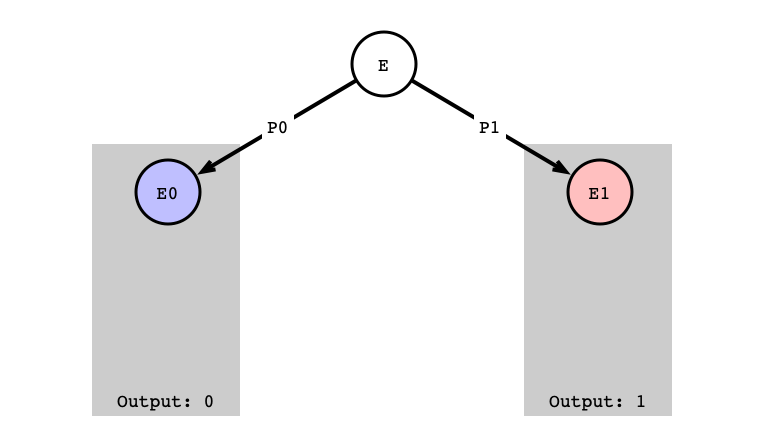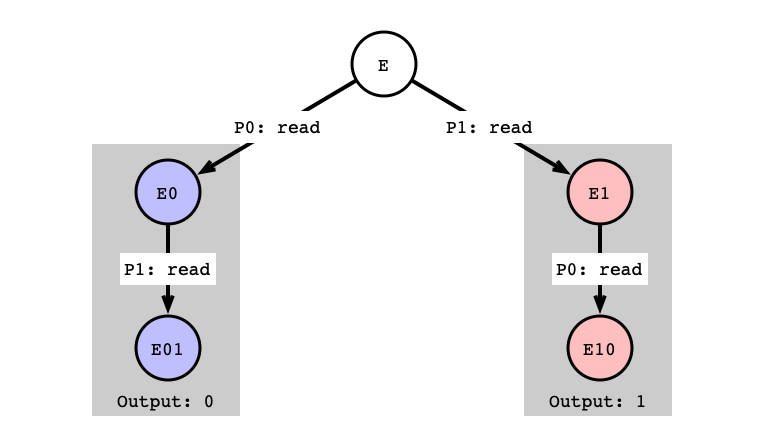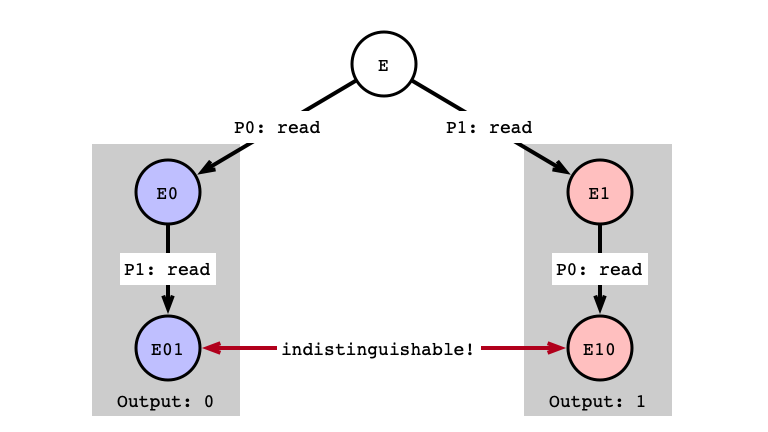Assumption:

• $P_0$’s next step is read
• $P_1$’s next step is write

• Start from critical state $E$
• if $P_0$’s read step is next, output is 0
• if $P_1$’s write step is next, output is 1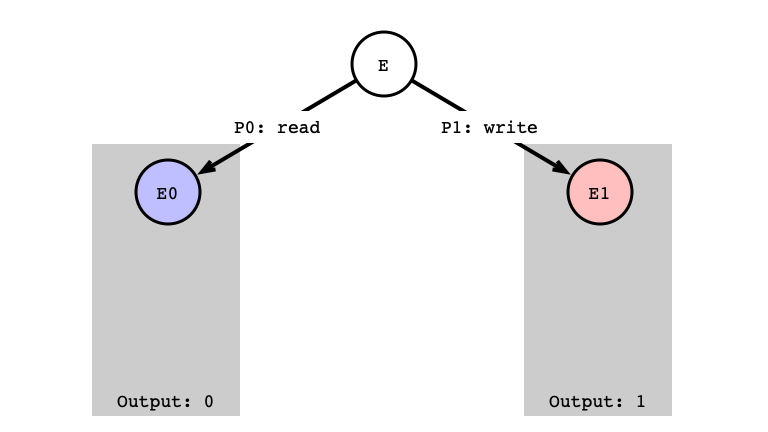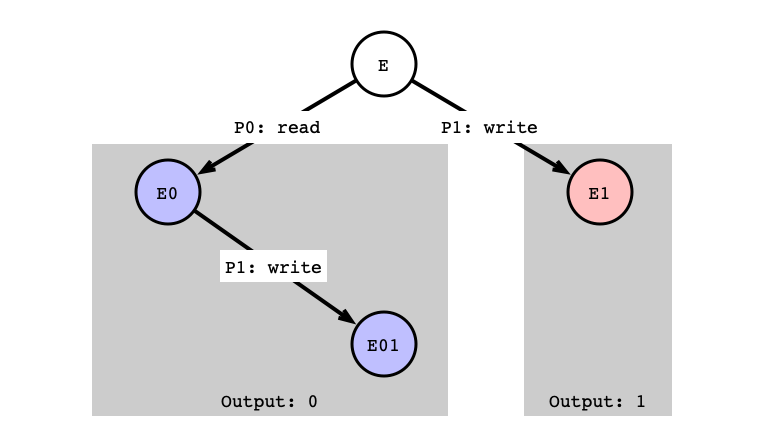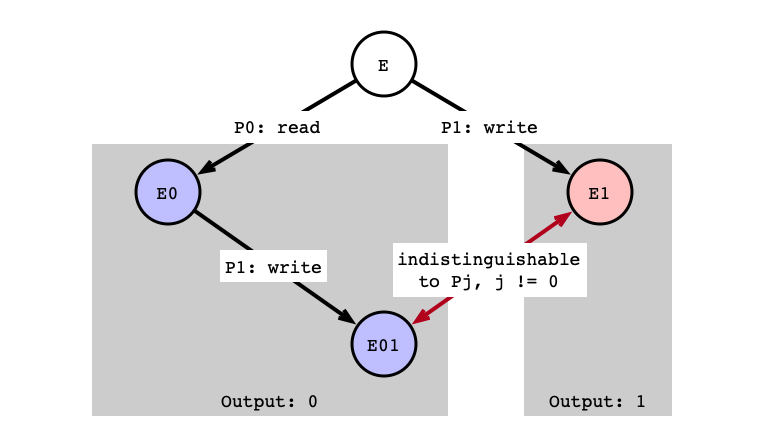read/write $P_0$ CrashesCase 3: write/write

Assumption: next operation for both $P_0$ and $P_1$ is write

Subcases:

• Sub-case a: write to different registers
• Sub-case b: write to same register

write/write Different Registers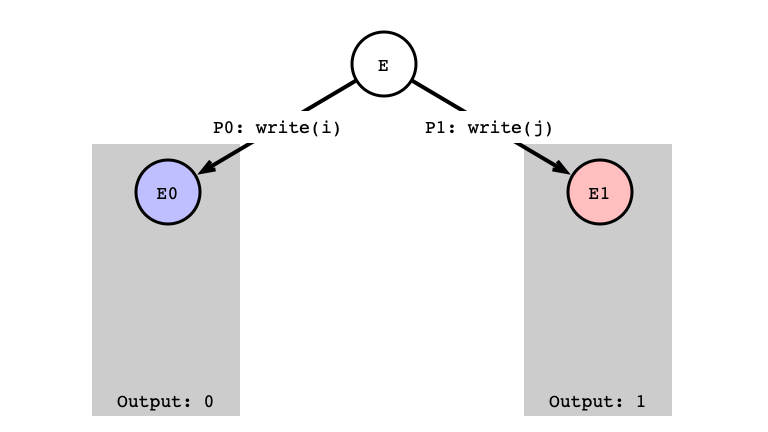write/write Next Step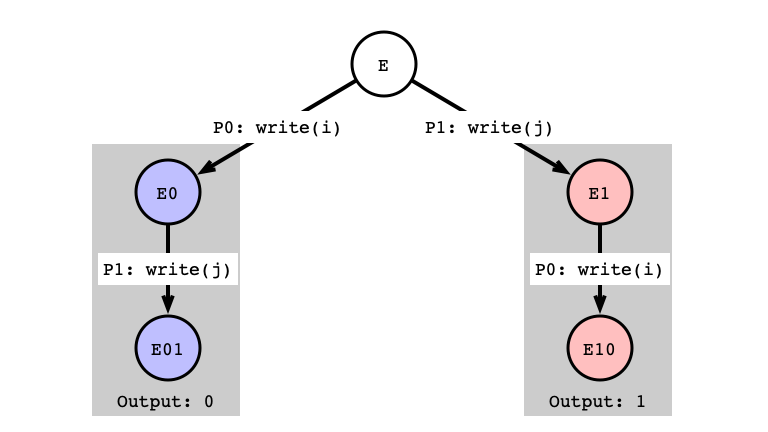write/write Indistinguishable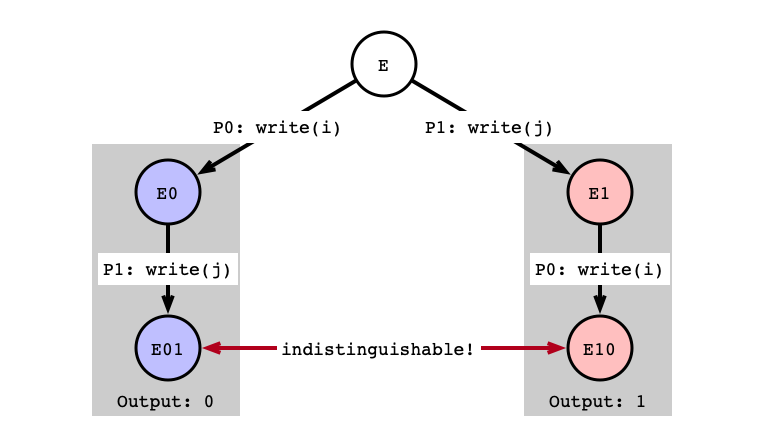write/write Same Register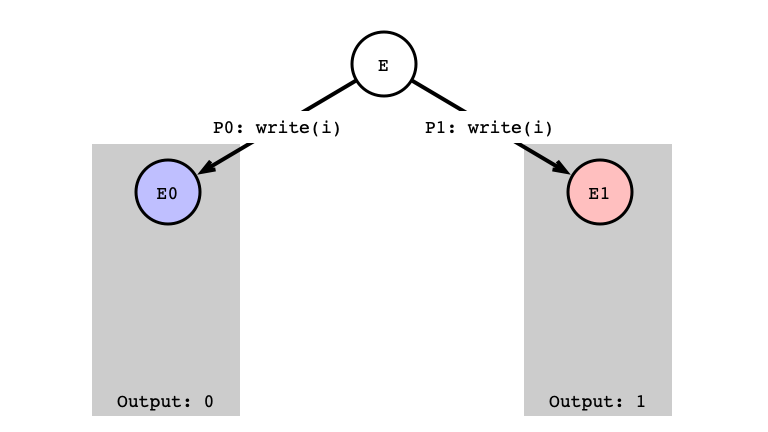write/write Next Step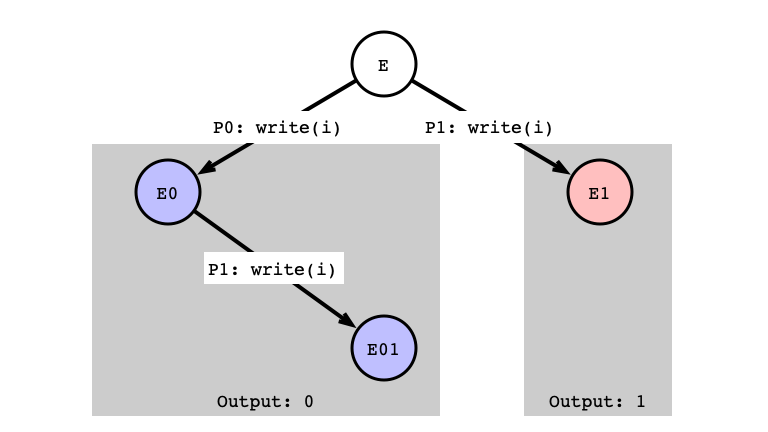write/write Indistinguishable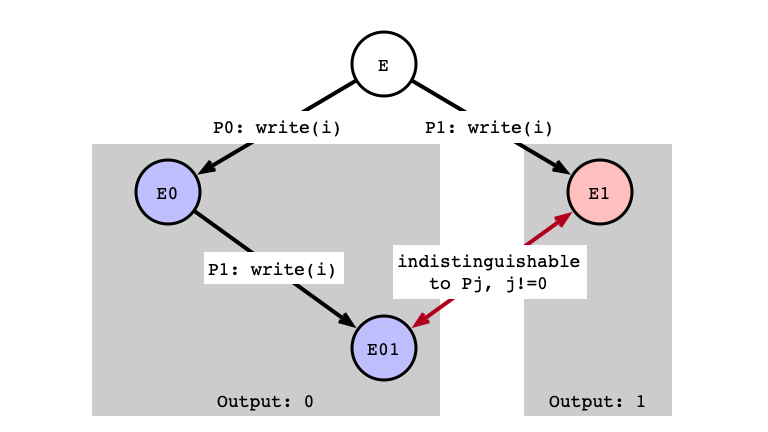Conclusion

In general:

• Indistinguishable executions produce same output

Assuming a wait-free consensus protocol using only read/write registers:

1. Showed there is a bivalent initial state
2. Showed there is a critical execution
3. Given a critical execution
• found indistinguishable states that must give different outputs

Remark. 1 and 2 hold for all protocols; 3 assumes only read/write registers

Consensus is Impossible?

Well not quite!

• We just proved impossibility in our computational model!
• wait-free (or faults)
• nasty scheduler!

Does the Model Reflect Reality?

• we have stronger primitives!
• compareAndSet
• we might have better schedulers
• round-robin/synchronous
• faults could be worse
• Byzantine faults

Implications

1. Atomic read/write registers are insufficient to solve fundamental tasks in parallel computing
• this drives the development of hardware primitives (e.g. CAS)
2. We can quantify the computational power of primitive operations
• read/write registers have consensus number 1
• FIFO queues have consensus number 2
• given a wait-free queue, 2 threads can solve consensus (How?)
• $\implies$ cannot implement concurrent queues with read/write registers
• Can use compareAndSet to achieve consensus (How?)

Coda

Four Morals

1. Parallelism is powerful
2. Communication is expensive
• cache locality and performance
3. Synchronization is subtle
• locks
• concurrent data structures
• impossibility (FLP)
4. Theory meets practice
• cannot reason about correctness/performance without understanding hardware